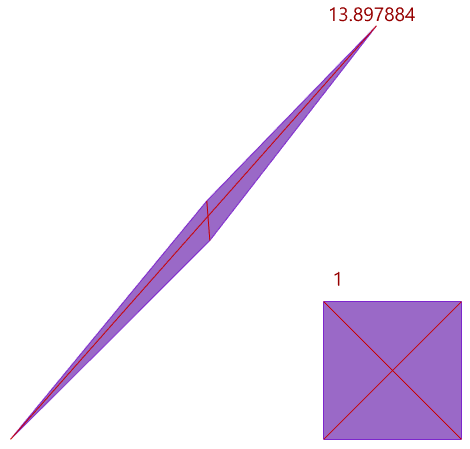In QuadrangulateMesh options Rhino 6 has the following options:
Planarity
Rectangularity
Increment

When I read associated help everything is clear but I have no clue why I should input any value into “Planarity” input instead of inputting “Rectangularity” only. Planarity is simply given by the information about neighbouring triangle faces in the original file - there is nothing to adjust here.

What in fact is the difference between the values to be input into these two windows?

And what are spinner controls?

Planarity The angle between the face normals of the triangles.
Rectangularity Specify a number greater than or equal to 1. If the adjacent triangles pass the planarity test then they will be merged into a quadrangle if the largest ratio of the lengths of the diagonal is less than or equal to the number you typed, then the triangles are converted to quadrangle.
Increment Increment value used by the spinner controls.

Regards

Hi -

The planarity setting allows you to tell Rhino what is needed to have 2 triangles be considered to be planar. This is not adjusting edges.

Once the planarity test is passed, the rectangularity test will decide if a pair of triangles actually gets converted to a quad.Spinner controls are the up and down arrows next to the numeric input fields.
If `Increment` is set to 1, pressing the `up` arrow will increase the value by 1. If it is set to 10, the value will increase by 10 for each mouse-click.
-wim

Thank you. But as I have already written, I still do not understand why two values should be input.

For example I decide that if 2 normals differ by 3 degrees, Rhino should make them planar.

This is planarity test. What exactly is rectangularity test? I’ve no idea what value I should input there.

On what ground is rectangularity test based?

Regards

Maybe the bigger angle for planarity test is set, the bigger tolerance should be set for rectangularity test

because it it impossible to keep common edges for all quadrangles (we get posts in the model instead of

a continuous surface).

Adjacent triangles will be merged into a quadrangle if the largest ratio of the lengths of the diagonal is less than or equal to the number you typed.

This is about the shape of the triangles. If you want to allow long and skinny quads, you’ll have to increase this value. The numbers in the following picture are the largest ratios of the lengths of the diagonals:-wim

Thank you, Wim.

Why can’t they just explain it in the help file.

Kind regards

MaciekHi @wim,

May just be me, but QuadrangulateMesh doesn’t seem to be autocompleting. Is it being hidden by design? That functionality seems sort of tucked into Mesh2. Is it redundant?

In WIP 7.0.20133.11585, 12/05/2020. At first I thought it had maybe just gone but I typed it in regardless (as QuadRangulatemesh)

Hi Jonathan -

Yes, it is.
Quoting from RH-54821:

Users are getting confused between the old QuadrangulateMesh and the new QuadRemesh commands. We feel QuadRemesh is much better and that’s what will make users the happiest.

-wim